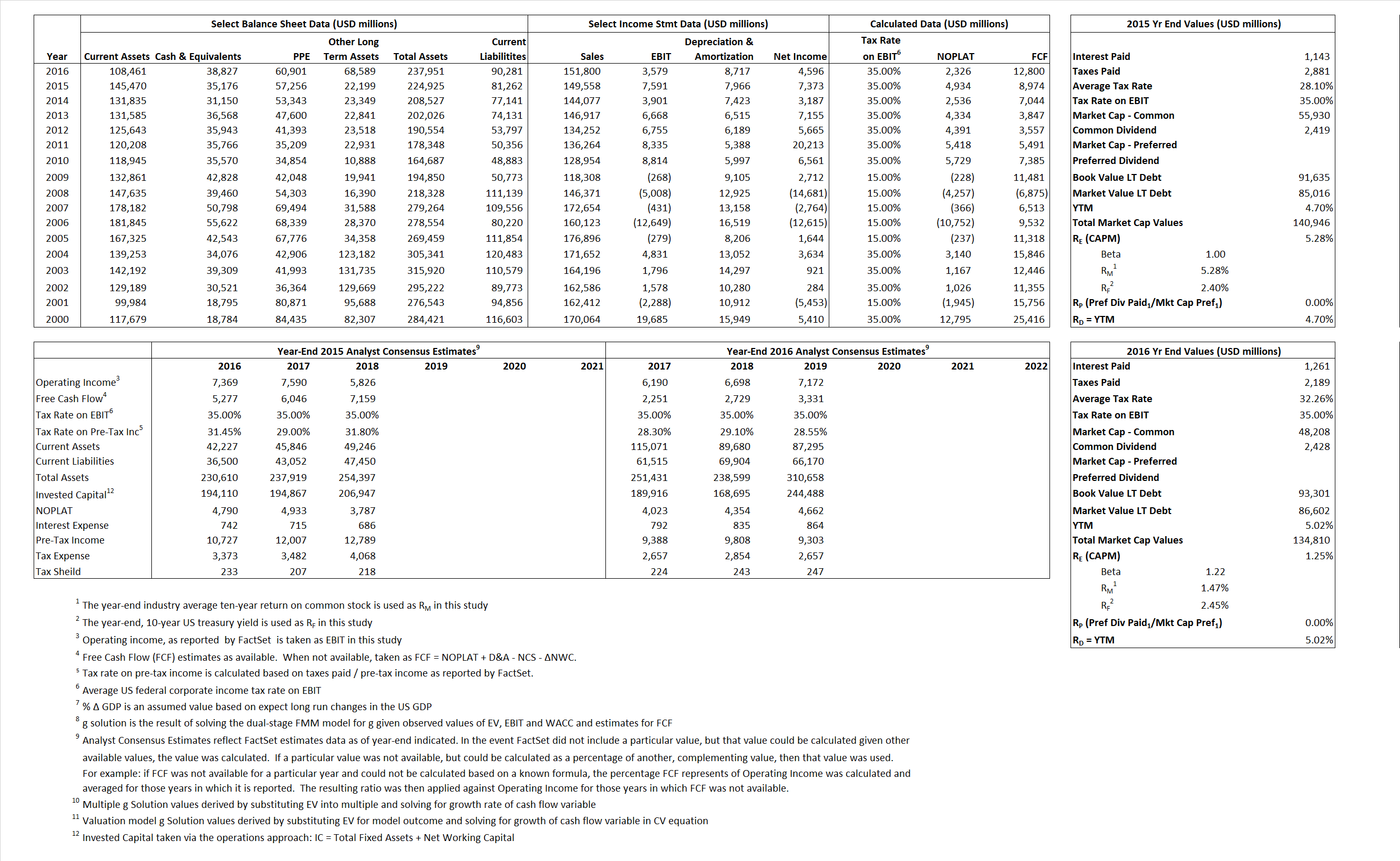# Ford Motor

## Analyst Listing

The following analysts provide coverage for the subject firm as of May 2016:

 Broker Analyst Analyst Email KeyBanc Capital Markets Brett D. Hoselton bhoselton@key.com Morningstar David Whiston david.whiston@morningstar.com Daiwa Securities Co. Ltd. Jairam Nathan jairam.nathan@us.daiwacm.com Buckingham Research Joseph C. Amaturo jamaturo@buckresearch.com RBC Capital Markets Joseph Spak joseph.spak@rbccm.com Susquehanna Financial Group Matthew T. Stover matthew.stover@sig.com CRT Capital Group Michael Ward mward@sterneageecrt.com Eugene Investment & Securities Moon-Su Chang moonsu.chang@eugenefn.com Deutsche Bank Research Rod Lache rod.lache@db.com Craig Hallum Capital Group Steve L. Dyer sdyer@craig-hallum.com Argus Research William Selesky bselesky@argusresearch.com

## Primary Input Data## Derived Input Data

### Equational Form

Net Operating Profit Less Adjusted Taxes NOPLAT 4,934  2,326$NOPLAT\, =\, EBIT\, x\, (1 \,-\, Avg \,\,Tax\,\, Rate\,\, on\,\, EBIT)$
Free Cash Flow FCF 8,974 12,800$FCF\,=NOPLAT\,+\,Non-Cash\,Expenses-\Delta NWC\,-\,NCS$
Tax Shield TS 321 407$TS\,=\,Interest\,\,Paid\,\,x\,\, Avg \,\,Tax\,\,Rate\,\, on\,\, Pre-Tax\,\, Income$
Invested Capital IC 143,663 147,670$IC\,=\,Fixed\,\,Operating\,\,Assets\,\,+\,\,Net\,\, Working\,\, Capital$
Return on Invested Capital ROIC 3.43% 1.58%$ROIC\,=\,\frac { NOPLAT }{ IC }$
Net Investment NetInv  20,243 12,724$NetInv\,=\,{ {IC}_{1}}-{{IC}_{0}}+Depreciation$
Investment Rate IR 410.26% 546.95%$IR\,=\,\frac {NetInv}{NOPLAT}$
Weighted Average Cost of Capital
WACCMarket 4.13% 2.63%$WACC\,=\,\frac { E }{ V } { R }_{ E }\,+\,\frac { P }{ V } { R }_{ P }\,+\,\frac { D }{ V } { R }_{ D }\left( 1- Avg\,\, Tax\,\,Rate\,\,on\,\,Pre-Tax\,\,Income \right)$
WACCBook   4.30%   4.08%
Enterprise value
EVMarket  105,770  95,983$EV\,=\,Market\,\,Cap\,\,Equity\,+\,\,Long\,\,Term\,\,Debt\,-\,Cash$
EVBook  112,389  102,682
Long-Run Growth
g = IR x ROIC
14.09%    8.62% Long-run growth rates of the income variable are used in the Continuing Value portion of the valuation models.
g = %$\Delta$ GDP    2.50%    2.50%
Margin from Operations M   5.05%   2.36%$M\,\,=\,\,\frac{EBIT}{SALES}$
Depreciation/Amortization Rate D   51.21%   70.89%$D\,\,=\,\,\frac{D+A}{EBITDA}$

## Valuation Multiple Outcomes

The outcomes presented in this study are the result of original input data, derived data, and synthesized inputs.

### model g solution

12/31/2015 12/31/2016 12/31/2015 12/31/2016 12/31/2015 12/31/2016

EV/SALES$\frac {EV}{Sales} \,= \,\frac{ROIC\, -\, g}{ROIC\,(WACC\,-\,g)}\,(1\,-\,T)\,(M)$

0.71  0.63  1.49%  -.39%  1.82%  -0.92%

EV/EBITDA$\frac {EV}{EBITDA} \,= \,\frac{ROIC\, -\, g}{ROIC\,(WACC\,-\,g)}\,(1\,-\,T)\,(1\,-\,D)$

6.80 7.81  1.49% -.39%  1.82% -0.92%

EV/NOPLAT$\frac {EV}{NOPLAT} \,= \,\frac{ROIC\, -\, g}{ROIC\,(WACC\,-\,g)}$

21.44  41.26  1.49% -.39%  1.82% -0.92%

EV/FCFOPS$\frac {EV}{FCF_{OPS}} \,= \,\frac{ROIC\, -\, g}{ROIC\,(WACC\,-\,g)}\,(1\,-\,T)$

11.79 7.50  1.49% -.39%  1.82% -0.92%

EV/EBIT$\frac {EV}{EBIT} \,= \,\frac{ROIC\, -\, g}{ROIC\,(WACC\,-\,g)}\,(1\,-\,T)$

13.93 26.82  1.49% -.39%  1.82% -0.92%

EV/IC$\frac {EV}{IC} \,= \,\frac{ROIC\, -\, g}{WACC\,-\,g}$

0.74 0.65  1.49% -.39%  1.82% -0.92%